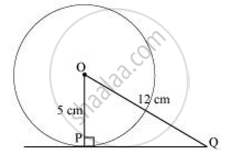Share

Books Shortlist

A tangent PQ at a point P of a circle of radius 5 cm meets a line through the centre O at a point Q so that OQ = 12 cm. Length PQ is : - CBSE Class 10 - Mathematics

Question

A tangent PQ at a point P of a circle of radius 5 cm meets a line through the centre O at a point Q so that OQ = 12 cm. Length PQ is :

• 12 cm.

• 13 cm

• 8.5 cm

• sqrt119 cm test

Solution

We know that the line drawn from the centre of the circle to the tangent is perpendicular to the tangent.

∴OP ⊥ PQ

By applying Pythagoras theorem in ΔOPQ,∴OP+ PQ= OQ

52 + PQ=12

PQ=144 − 25

PQ = sqrt119

Hence, the correct answer is sqrt119 cm test.

Is there an error in this question or solution?

Video TutorialsVIEW ALL 

Solution A tangent PQ at a point P of a circle of radius 5 cm meets a line through the centre O at a point Q so that OQ = 12 cm. Length PQ is : Concept: Tangent to a Circle.
S